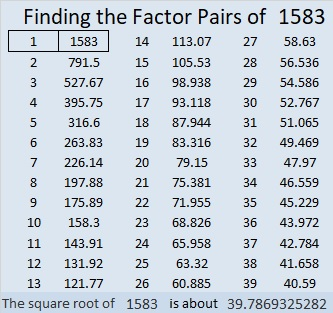# 1583 The Logic for This Mystery Level Puzzle

Contents

### Today’s Puzzle:

The logic for this mystery level puzzle starts out simple, but gets more complicated as you go along. Will you figure out where to put the numbers from 1 to 10 in both the first column and the top row so that those numbers are the factors of the given clues?### Factors of 1583:

• 1583 is a prime number.
• Prime factorization: 1583 is prime.
• 1583 has no exponents greater than 1 in its prime factorization, so √1583 cannot be simplified.
• The exponent in the prime factorization is 1. Adding one to that exponent we get (1 + 1) = 2. Therefore 1583 has exactly 2 factors.
• The factors of 1583 are outlined with their factor pair partners in the graphic below.How do we know that 1583 is a prime number? If 1583 were not a prime number, then it would be divisible by at least one prime number less than or equal to √1583. Since 1583 cannot be divided evenly by 2, 3, 5, 7, 11, 13, 17, 19, 23, 29, 31, or 37, we know that 1583 is a prime number.

### More about the Number 1583:

1583 is the sum of two consecutive numbers:
791 + 792 = 1583.

1583 is also the difference of two consecutive squares:
792² – 791² = 1583.

What do you notice about the consecutive numbers in those two facts?

This site uses Akismet to reduce spam. Learn how your comment data is processed.